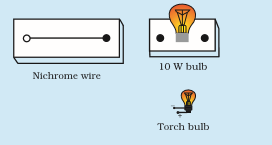# What is electrical resistivity of a material? What is its unit? Describe an experiment to study the factors on which the resistance of conducting wire depends.

Resistivity is an inherent property of a conductor which resists the flow of electric current. The resistivity of each material is unique. SI unit of resistance is Ωm.

## Experiment to study the factors on which the resistance of conducting wire depends.

Take a nichrome wire, a torch bulb, a 10 W bulb and an ammeter (0 – 5 A range), a plug key and some connecting wires.

• Set up the circuit by connecting four dry cells of 1.5 V each in series with the ammeter leaving a gap XY in the circuit, as shown in Figure.
• It is observed that resistance depend on the material of the conductor
• Length of conductor determines the resistance
• Resistance depends on the area of cross-section.• Complete the circuit by connecting the nichrome wire in the gap XY. Plug the key.
• Note down the ammeter reading. Take out the key from the plug.
• Replace the nichrome wire with the torch bulb in the circuit and find the current through it by measuring the reading of the ammeter.
• Now repeat the above step with the 10 W bulb in the gap XY.
• Are the ammeter readings different for different components connected in the gap XY? What do the above observations indicate?
• You may repeat this Activity by keeping any material component in the gap. Observe the ammeter readings in each case. Analyse the observations.(22)(15)